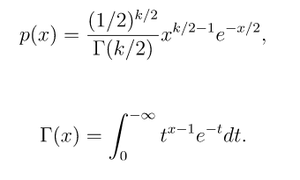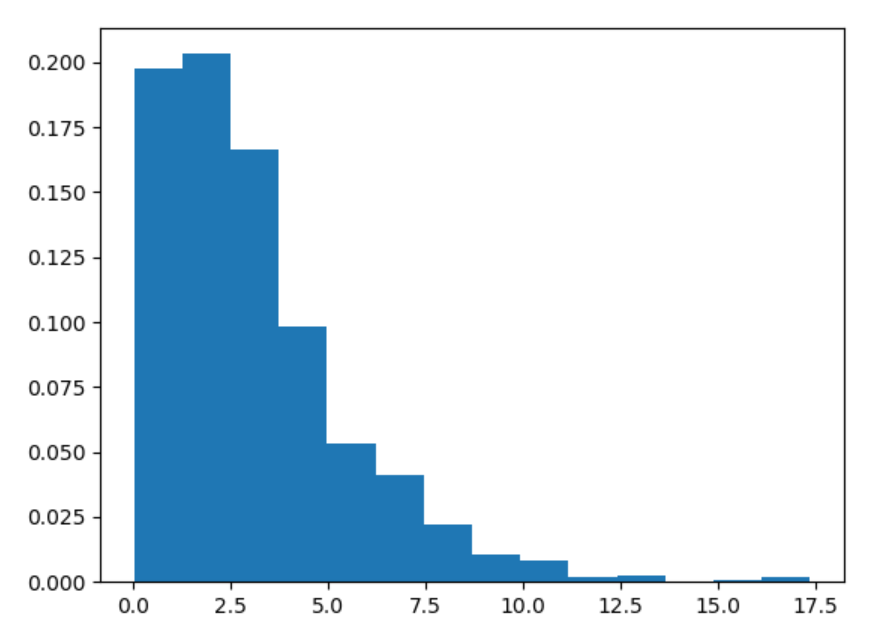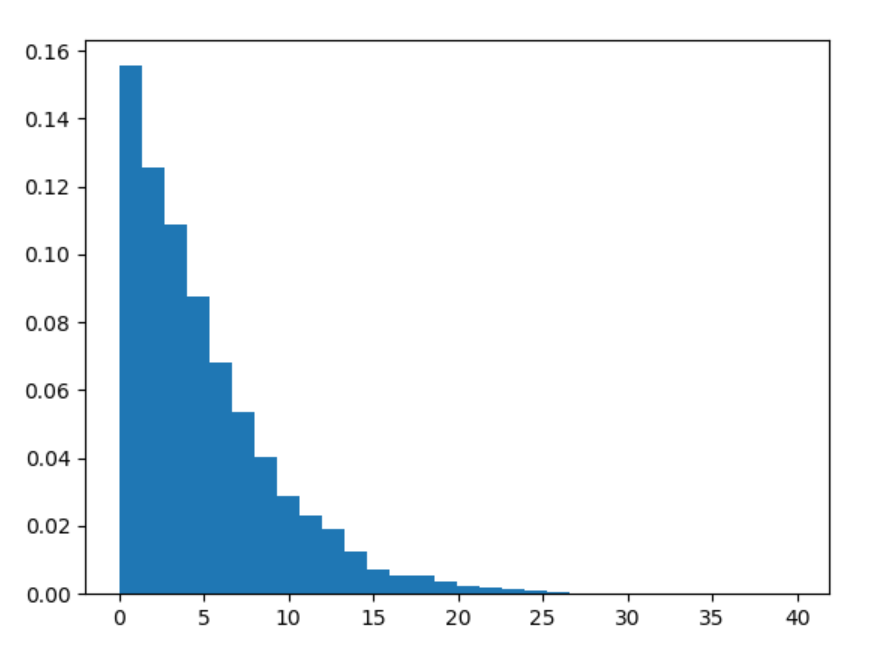# numpy.random.chisquare() in Python

• Last Updated : 15 Jul, 2020

With the help of chisquare() method, we can get chi-square distribution by using this method. Mainly we can use this distribution in hypothesis testing.chi-square distribution

Syntax : numpy.random.chisquare(df, size=None)

Attention geek! Strengthen your foundations with the Python Programming Foundation Course and learn the basics.

To begin with, your interview preparations Enhance your Data Structures concepts with the Python DS Course. And to begin with your Machine Learning Journey, join the Machine Learning - Basic Level Course

Parameters :

1) df – number of degree of freedom and must be >0.

2) size – Output shape of scalar array.

Return : Return the scalar numpy array.

Example #1 :

In this example we can see that by using chisquare() method, we are able to get the chi-square distribution and return the scalar numpy array by using this method.

## Python3

 `# import chisquare``import` `numpy as np``import` `matplotlib.pyplot as plt`` ` `# Using chisquare() method``gfg ``=` `np.random.chisquare(``3``, ``1000``)`` ` `count, bins, ignored ``=` `plt.hist(gfg, ``14``, density ``=` `True``)``plt.show()`

Output :Example #2 :

## Python3

 `# import chisquare``import` `numpy as np``import` `matplotlib.pyplot as plt`` ` `# Using chisquare() method``gfg ``=` `np.random.chisquare(``5``, ``10000``)``gfg1 ``=` `np.random.chisquare(gfg, ``10000``)`` ` `count, bins, ignored ``=` `plt.hist(gfg1, ``30``, density ``=` `True``)``plt.show()`

Output :My Personal Notes arrow_drop_up